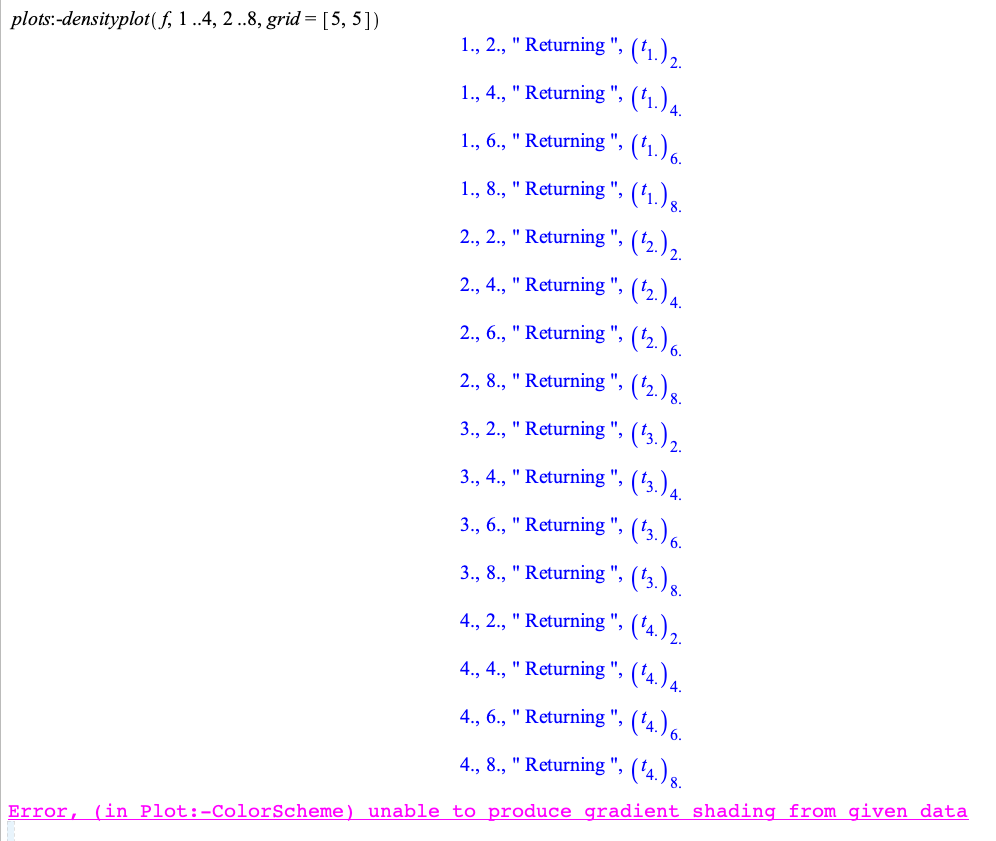# Question:Why can't one access certain decimal keys in tables?

## Question:Why can't one access certain decimal keys in tables?

Maple 2022

This question is about an aspect of the code below. In fact, the code could be made simpler to get at the question, but I chose to show you how the problem originated.

We start with a 4x3 matrix A. There is a procedure, convertArrayToTable, that takes this matrix and creates a table. The table is such that the keys are the numbers in the first column of A. For each such key k1, the associated value is another table. The latter table has as keys the values in the second column that have k1 in the first column.

Now, further below, if you are curious I write about why I am doing this (what the exact real problem is). For now, I am generating a matrix B which in is formed by the concatenation of A and 2A, along the column (ie, A on top of 2A).

So for this matrix B, the table T that is generated has, for example a key 1 and the associated value is a table with keys 0.2 and 0.400000000000000.

I wish to be able to access T[0.400000000000000] but this is not working and I don't know why.convertArrayToTable := proc(arr)
local m, t, i, c1, c2, c3:

m := ArrayTools:-Size(arr):
t := Table([]):

for i from 1 to m do:
c1 := arr[i,1]:
c2 := arr[i,2]:
c3 := arr[i,3]:

if not assigned(t[c1]) then:
t[c1] := table([ c2 = c3]):
else:
t[c1][c2] := c3:
end:
end:
print(t):

return t:
end:(1)(2)(3)(4)(5)(6)(7)

Why can't I access the following key?(8)(9)So now just a brief note on why I want to create such a table.

I want to create a density plot based on the values in the rows of B. In the case of the simple example shown above, the grid of values would be based on values of x from 1 to 4 and of y from 0.2 to 0.400000000000000. Then I would specify, say "grid=[5,3]" for an eight point grid.

In this example I am assuming the points used by the densityplot procedure would be exactly the ones in the rows of B, ie (1,0.2), (2,0.2), (3,0.2), (4,0.2),(1,0.400000000000000),(2,0.400000000000000),(3,0.400000000000000),(4,0.400000000000000).

The function I would pass in the signature

densityplot(f, a..b, c..d)

would be a custom procedure that I am assuming would be called as f(x,y) and would simply look up T[x][y] in the table I created to get the value.

Of course in my real problem the grid has way more points.

I am making a lot of assumptions about this densityplot procedure, but the documentation isn't very clear at all.

Here is a proof of concept of what I am trying to achieve (note that below, I am avoiding the issue of the decimal keys in tables by using integers instead):convertArrayToTable := proc(arr)
local m, t, i, c1, c2, c3:

m := ArrayTools:-Size(arr):
t := Table([]):

for i from 1 to m do:
c1 := arr[i,1]:
c2 := arr[i,2]:
c3 := arr[i,3]:

if not assigned(t[c1]) then:
t[c1] := table([ c2 = c3]):
else:
t[c1][c2] := c3:
end:
end:
print(t):

return t:
end:(1)(2)(3)

f := proc(x,y)
global T:
print(x,y," Returning ",T[x][y]):
return T[x][y]:
end:In the above output, the output from the print statement in the f procedure is not being shown. Locally on my computer it is shown and is called at the expected values (the ones in the rows of B). So that is all good. The issue seems to always go back to keying in to tables with certain (but not all) decimal numbers